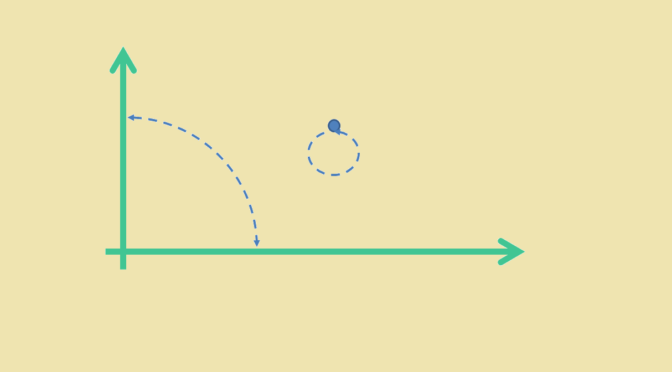Non linear map preserving orthogonality

Let $$V$$ be a real vector space endowed with an inner product $$\langle \cdot, \cdot \rangle$$.

It is known that a bijective map $$T : V \to V$$ that preserves the inner product $$\langle \cdot, \cdot \rangle$$ is linear.

That might not be the case if $$T$$ is supposed to only preserve orthogonality. Let’s consider for $$V$$ the real plane $$\mathbb R^2$$ and the map $\begin{array}{l|rcll} T : & \mathbb R^2 & \longrightarrow & \mathbb R^2 \\ & (x,y) & \longmapsto & (x,y) & \text{for } xy \neq 0\\ & (x,0) & \longmapsto & (0,x)\\ & (0,y) & \longmapsto & (y,0) \end{array}$

The restriction of $$T$$ to the plane less the x-axis and the y-axis is the identity and therefore is bijective on this set. Moreover $$T$$ is a bijection from the x-axis onto the y-axis, and a bijection from the y-axis onto the x-axis. This proves that $$T$$ is bijective on the real plane.

$$T$$ preserves the orthogonality on the plane less x-axis and y-axis as it is the identity there. As $$T$$ swaps the x-axis and the y-axis, it also preserves orthogonality of the coordinate axes. However, $$T$$ is not linear as for non zero $$x \neq y$$ we have: $\begin{cases} T[(x,0) + (0,y)] = T[(x,y)] &= (x,y)\\ \text{while}\\ T[(x,0)] + T[(0,y)] = (0,x) + (y,0) &= (y,x) \end{cases}$